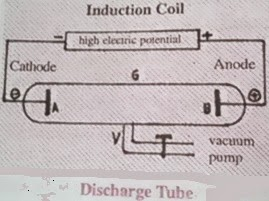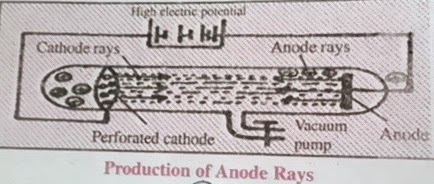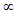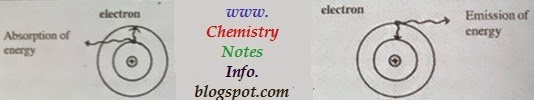## Atomic Structure

### What is an atom?

In this chapter we learn about atom, atomic structure, discovery of electron proton & neutron and many more interesting facts about atom.

Matter found around us in nature (like iron rod, glass, cup, pen, pencil etc.) is made up from very small particles, which is known as atoms.
we also says that "atoms are very small particles which made matter" so atom is fundamental unit of atom.

Greek Philosopher, Democritus describe atom as very small indivisible particle.

=> Listen This Basic Chemistry Podcast on Atomic Structure

'New System of Chemical Philosophy' is a book about atom written by John Dalton in 1703.

## Dalton Atomic Theory

According to this theory atom cannot be divided, but after some years it is proved that atom can be divided further by many scientists like J.J.Thomson, L.Rutherford, N.Bohr, Chadwick etc.

### Constituting particles of atom

Atom made up from 3 main particles-
1. Electron 2. Proton 3. Neutron

## Discovery of Electron

Sir J.J.Thomson and W.Crooks did many experiments with discharge tube for the discovery of electron.
Discharge tube have tube like shape made from glass with two electrodes (Cathode -ve and Anode +ve) in vacuum created by vacuum pump connected to discharge tube. High electric potential is applied between two electrodes.Discharge Tube
Air is bad conductor of electricity so vacuum pump is connected to reduce pressure to 0.02mm inside discharge tube currents starts flowing between electrodes and light is emitted. On further reducing pressure in discharge tube greenish yellow color fluorescence occur. As these rays emerging from cathode, Sir J.J.Thomson named them as cathode rays.
Deflection of cathode rays towards positively charged plate in electric field proves that these rays carry negatively charged particles.
These negatively charged particles are named as electrons.

### Properties of Cathode Rays

1. Cathode rays always travel in straight line.Production of Cathode Rays
2. Velocity of cathode rays and velocity of light are approximately equal.
3. On applying electric field in the path of cathode rays, cathode rays turn towards +vely charged plate that proves cathode rays are made up from negatively charged particles.
4. Cathode rays rotate light wheel placed in their path that proves cathode rays are made from particles having mass.
5. Cathode rays pass through thin metal foil and it gets slightly heated up by action.
6. These rays produce fluorescence at walls of glass tube.
7. Cathode rays ionize gases and also affect photographic plate.
8. When these rays strike any metal with high melting point (like tungsten W) they produces X-Rays.

### What is X-rays?

X-rays are electromagnetic radiation , X-rays was discovered by W.K.Roentgen in 1895. X-rays are also known as Roentgen rays.

### Why X-rays are used in medical sciences?

X-rays are used in medical sciences because x-rays have high penetrating power.

### Determination of charge to mass ratio of electron (e/m)

J.J.Thomson conduct many experiments that charge to mass ratio of an electron remains same, irrespective of nature of gas and nature of cathode electrode material.
value of e/m = -1.76x108 coulombs/gram

### Millikan's Oil Drop Experiment

Millikan's Oil Drop Experiment or Determination of charge of electron is conduct by an American Scientist R.A.Millikan, who perform an experiment on the charge on oil drops. R.A.Millikan perform several experiments to calculate charge on oil drops and he gets every time its value equal to -1.6x10-19 coulomb.When these results associated with results of cathode rays then conclude that charge present on particle of cathode rays is -1.6x10-19 coulomb.

#### Calculation of mass of electron

As we  know e/m = -1.76x108 coulombs/gram
e = -1.6x10-19 coulomb
then,
(e/m)/e = (-1.76x108)/(-1.6x10-19)
so,
m = 9.102x10-28 gram
m = 9.102x10-31 kilogram

#### Mass of electron in comparison with atom

Mass of electron in comparison with atom is described below-
Mass of 1 mole of Hydrogen = 1.008gms
Number of hydrogen atom in 1 mole = 6.023x1023
Mass of 1 atom of hydrogen = 1.008/6.023x1023
= 1.67x10-27kg
Mass of electron is 9.109x10-31
then,
=  Mass of 1 atom of hydrogen/Mass of electron
= (1.67x10-27)/(9.109x10-31) = 1837
so,
Mass of an electron is 1/1837 th the mass of a hydrogen atom.

## Discovery of Proton

As we know electron is negatively (-vely) charged particle but atom is electrically neutral so there should be some particles which have positive (+ve) charge to neutralize negative (-ve) charge electron.
In 1886, a German scientist E.Goldstein established the presence of +vely charged particles. These positively charged rays travel from anode to cathode so called as anode rays or positive rays.

#### Why anode rays are called as canal rays?

Anode rays passes through canals or perforation in cathode so called as canal rays.

### Properties of Anode Rays

1. Anode rays always travel in straight line.
2. Anode rays rotate light wheel placed in their path that proves anode rays are made from particles having mass.Which produces mechanical action.
3. On applying electric field in the path of anode rays, anode rays turn towards -vely charged plate that proves that anode rays are made up from positively charged particles.Production of Anode Rays

### Determination of charge to mass ratio of proton (e/m)

On the basis of many experiments performed for anode rays, scientist Wein concluded that charge to mass ratio of proton changes with change in nature of gases present in discharge tube. This value (e/m) is maximum for hydrogen gas = 9.58x104 coulomb per gram. "Positively charged particle of hydrogen is fundamental particle of matter that is called proton."

#### Charge on proton

Charge on proton is equal to charge on electron but is of opposite nature.
Charge on proton = 1.602x10-19 coulomb.
Mass of the proton
For hydrogen gas,
e/m = 9.58x104 coulomb per gram
charge of electron = 1.602x10-19 coulomb
so,
m = (e)/(e/m) = (1.602x10-19)/(9.58x104)
also,
m = 1.67x10-24 gram
m = 1.67x10-27 kg
so,
mass of proton is 1837 times more than mass of electron and is equal to mass of an hydrogen atom.

## Discovery of Neutron

Mass of atom is more than the mass of total proton and electron present in atom, which suggest the presence of another particle in atom which lead the discovery of neutron.
Chadwick in 1932 discover neutral particles of mass equal to mass of proton by bombarding beryllium metal with stream of fast moving particles through cyclotron. These particle are neutral in nature so named as neutron.
Mass of neutron = 1.6748x10-27 kg

#### There is three fundamental particles in atom

These fundamental particles are 1. Electron 2. Proton 3. Neutron

Many atomic models are proposed from time to time to show the actual structure of atom, some of them are described below-
1. Thomson's atomic model
2. Rutherford's atomic model
3. Bohr's atomic model

## Thomson's Atomic ModelThomson's Atomic Model
Thomson's atomic model is first atomic model proposed in 1898 related to atomic structure. This model is similar to water-melon in which positive charge protons is like pulp in which negative charge electrons like seeds in water-melon, also known as pudding model. This model is called Thomson's atomic model.

#### Drawback of Thomson's atomic model

Thomson's atomic model is unable to explain scattering experiment of Rutherford.

## Rutherford Model of Atom

This experiment is also known as alpa () particle scattering experiment. Rutherford bombard alpha particle on gold foil of thickness 0.0004cm and found that-
1. So many particles passes through the gold foil without any deflection.
2. Some alpha particle are deflected at different angles.
3. Very few about 1 in 20,000 are bounced back (at 360 degree) from gold foil.Rutherford Atomic Model
Rutherford concluded nuclear model of atom as-
1. Most of the part of the atom is hollow and neutral so alpha particles passes straight without any deflection.
2. All positive charge is present in center of atom so alpha particle are deflected at different angles by repulsion from positively charged center known as nucleus.
3. Only very few particles bounced back so size of nucleus is very small as compared to size of atom.
4. Electron moves around nucleus like planet moves around sun in orbits.
5. Mass of electron is negligible so all mass of atom is present in nucleus.
6. Atom is electrically neutral so number of electron is equal to number of proton present in atom.

#### Defects of Rutherford Atomic Model

1. Stability of atom is not explained on the basis of this model.
2. This model could not explain discrete spectrum.

## Bohr's Atomic Model

Neils Bohr in 1913 gives a simple model for atomic structure based on the quantum theory.
Main assumption of Bohr atomic model-
1. All atoms consist of dense, very small, positively charged nucleus that have all protons and neutrons in it.
2. Electron revolve around nucleus in definite energy paths known as orbits, shell or energy levels.
3. Orbits denoted by (n). value of n is whole number 1,2,3,4.......... etc. which are represented as K,L,M,N............. etc. respectively.Bohr Atomic Model
4. As we increase the value of n the orbit move farther from nucleus (means distance between nucleus and orbit of higher n is more than smaller n) and their energy also increases so n=1 or K shell have lowest energy.
5. If an electron revolve in same energy level then their is no change in its energy level.
6. As electron absorb energy from outside, it gets exited and move to higher energy level and come back after emitting energy to lower energy level.Absorption and Emission of Electron Energy

### Atomic Number

Number of protons present in nucleus of atom of an element is known as atomic number.
It is denoted by (z)
example : Carbon have 6 protons so its atomic number is 6 (z = 6)
For neutral atom,
atomic number (z) = number of protons (p) = number of electron (e)

Number of protons in cation > Number of electrons in cation
Number of protons in anion < Number of electrons in anion
Number of protons in neutral atom = Number of electron in neutral atom

### Mass Number

Mass number is also known as atomic mass. Atomic number or mass number is equal to number of protons and neutrons in atom.
Atomic mass = number of protons + number of neutrons
A = p+n
example : Helium have two protons and two neutrons so its atomic mass is 2+2=4

### Isotopes

Atoms of same elements which have same atomic number but different mass number is called isotopes.
example : Protium, Deutrium, Tritium are isotopes of hydrogen.

#### Types of Isotopes

1. Non-radioactive isotope example C(12), C(13)
2. Radioactive isotopes example U(235), U(233), U(238), U(239)

### Isobars

Atoms of different elements which have similar atomic mass and different atomic number are called isobars.
example : Ca(40), Ar(40), Kr(40)
50Cr24, 50Ti22
Isobars do not similar in physical and chemical properties

#### Distribution of electrons in various orbits : ( Bohr Bury  Scheme )

In 1921, Bohr and Bury gives some laws for distribution of electrons in various orbits, which are called as Bohr-Bury Scheme.
Rules of Bohr Bury scheme are as follows-
1. Maximum number of electron in any shell should be 2n2 , where n is serial number of shell.
2. Maximum number of electron that exist in outermost shell or orbit of any atom is eight while maximum number is eighteen for penultimate shell.
3. According to this law, it is not necessary that another shell is formed after completion of 1st shell. A new shell is formed as number of electrons reaches eight in any orbit or shell.

### Valence Electrons and Valency

Outermost incomplete shell of any atom is known as Valence Shell and electron present in incomplete outermost shell are known as Valence Electrons , which participate in formation of bond.
• If atoms have 1 to 4 valence electrons then VALENCY = Number of valence electron
• If atoms have 5 to 8 valence electrons then VALENCY = 8 - Number of valence electron
• But these above rule is not applicable to atoms which show variable valency

Radioactivity is discovered by French scientist Becquerel in 1896. Substances which emits radiation is known as radioactive substances and this property of substances to emit radiation is called as radioactivity.

Rutherford conduct some experiments with radioactive substances and his observations are as follows-
1. Rays which turned toward negative electrode is known as alpharays, mass of alpha particles is 4 a.m.u.  and charge is 2 units of positive charge.
2. Rays which turned toward positive electrode is known as betarays, mass and charge of beta particle is equal to mass and charge of electron.
3. The rays which do not turned towards any electrode is known as gammarays, gamma rays are electromagnetic rays and not made from matter particle.

#### Penetrating Power

Gamma > Beta > Alpha
Gamma rays have maximum penetrating power while Alpha has lowest.

### Tracer technique of radioactive isotopes

As per requirement, small amount of radioactive isotopes is added to its element then many physical and chemical complex reaction are studied.

### Various fields of tracer techniques

1. In the field of chemical science.
2. In the field of medical science.
3. In the field of agriculture.
4. In the industrial fields.
5. And in other scientific fields.

#### Carbon Dating

In this technique carbon isotope is used to determine the age of rocks, minerals, fossils, dead animals and dead plants.